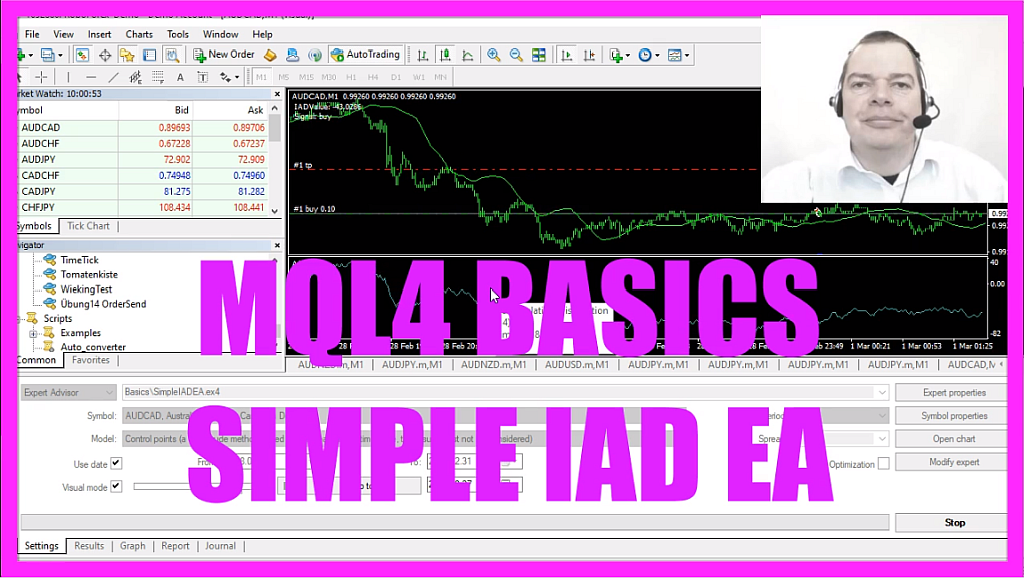In this video we are going to calculate this little accumulation distribution oscillator value and use it as a filter for a simple moving average, so let’s find out how to do that with mql4.
To start please click on the little button here or press F4 on your keyboard, now you should see the Metaeditor window and here we click on file, new, expert advisor from template, continue, I will call this file simple iad ea, click on continue, continue and finish.
Now you can delete everything above the on tick function and the two comment lines here, we start by creating a signal string, this one will be empty because we need to calculate the current signal, we also want to calculate a moving average that is done by using the ima function, in this case we calculate the simple moving average for twenty candles, we have done that before so maybe you want to watch the previous videos in this basic series and on top of that simple moving average I want to use the accumulation distribution oscillator as a filter, so let’s also calculate that one, that is done by using the iad function for the current symbol on the chart and for the currently selected period on the chart, the last value here is for a shift, we don’t use that because first, we want to calculate the value for the current candle zero but afterwards we want to calculate the accumulation distribution value for the candle ten candles before.
Now let’s create an entry condition.
First I want to know if the current ask price is above the moving average value, that is the case whenever the candles are above the green line here and on top of that I want to know if the current value for the oscillator is bigger than the last value for ten candles before, and that would be true whenever the oscillator values are rising and in that case we have a buy signal, so I assign the word buy to our signal, otherwise if we have a downtrend, that would be the case when the bid price is below the moving average so basically when the candles are below the green line here.
And I also want to know if the current accumulation distribution value is below the one for ten candles before, that is the case when the oscillator line goes down and if this is true I assign the word sell to our signal.
Now if the signal equals buy and orders total equals zero, that means that we have an uptrend, a buy signal and no open orders, we use the order send command to open a buy trade for ten micro lot and if the signal equals sell and we also have no open orders that’s when we use order send to open a sell trade for ten micro lot.
And finally we use the comment statement to output the current iad value followed by the calculated value and in a new line the signal that might be buy or sell and that’s about it.
Now if this all was too fast for you or if you don’t understand what is going on with all that code, maybe you want to watch the other videos in the basic series or maybe even the premium course is interesting for you, you can find that one on the website, for now, please click on the compile button or press F7, you shouldn’t get any errors here and if that is the case you can click on a little icon here or press F4 to go back to Metatrader.
And in Metatrader we want to use a little trick, first we click on insert, indicators, trend, moving average, here we pick a period of twenty candles, the shift value is zero, we want the simple moving average that is calculated based on the close price, so let’s click on ok, and here is the sma line, let’s repeat the same thing, this time insert, indicators, volumes, accumulation distribution, we go with the default values here so let’s click on ok, now your chart should look like this, so let’s save that for the strategy tester by right-clicking into the chart, please select template, save template. Now we want to save that file as tester dot tpl, you can override the old one.
To start the backtest please click on view, strategy tester or press control and r, pick the new file, simple iad ea dot ex4, mark the visual mode here and start a test.
And here is our little expert advisor at work, it produces buy and sell signals based on the values of the simple moving average and the accumulation distribution oscillator and you have coded it yourself with a few lines of mql4 code.